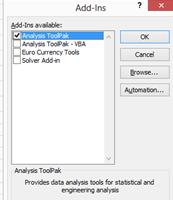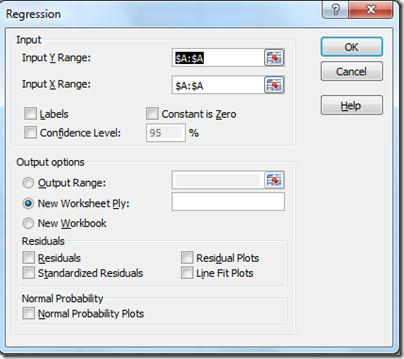### Regression Analysis In Excel

Regression analysis is a statistical tool which is used for analyzing and modeling variables, it lets you see how value of one variable (dependent) changes when any one of the other independent variables changes. It helps us to understand the relationship between a dependent variable and one or more independent variables.

In other words Regression is a method of estimating the portion of a cost that is variable and the portion that is fixed. The following tutorial will help you use Excel to “run” a regression.

### Accessing Regression Analysis in Microsoft Excel

Go to File > Excel Options > Add-Ins > click Analysis ToolPak > then click GOFollowing dialog box will be open, Click Analysis Toolpak and then click OK.Data Analysis button will appear on Data ribbon. Under Data Analysis feature Regression function can be found.Once Data Analysis is enabled, open it and choose the Regression and click ok button.• Input Y Range option: Select dependent variables
• Input X Range option: Select independent variables.
• Output options specify how you would like the results to be displayed,
• Residuals contain options to draw the results as charts.

For practice type X & Y values in the following style:Once you click the Ok button following regression table will be created in new sheet as follows.## Template

```Further reading: